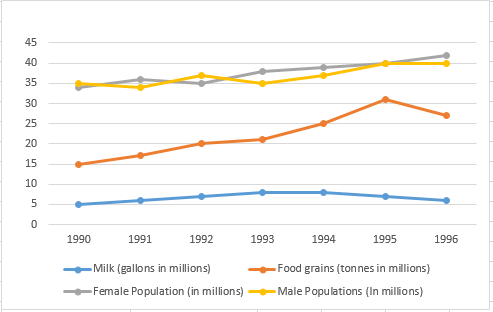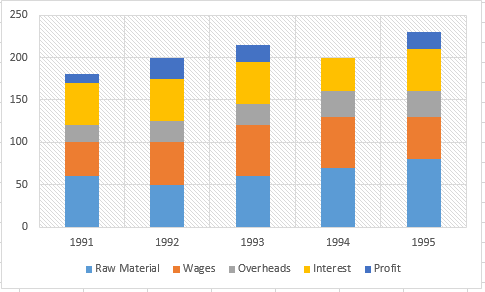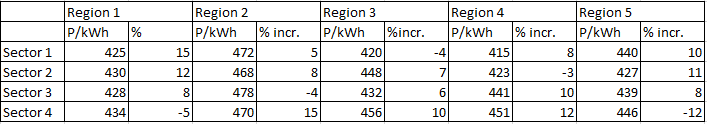# CAT 1997

Instructions

The graph given below shows the quantity of milk and food grains consumed annually along with female and male population (in millions). Use the data to answer the questions that follow.Question 11

# Referring to the previous question, in which year was the per capita consumption of this nutrient highest?

Instructions

The graph given below gives the yearly details of money invested in producing a certain product over the years 1991 to 1995. It also gives the profit (in ‘000 rupees).Question 12

Question 13

Question 14

Question 15

Question 16

Question 17

# If the interest component is not included in the total cost calculation, which year would show the maximum profit per unit cost?

Instructions

The following table gives the tariff [in paise per kilo-watt-hour (kWh)] levied by the UPSEB in 1994-95, in four sectors and the regions within them. The table also gives the percentage change in the tariff as compared to 1991-92.Question 18

Question 19

Question 20

OR